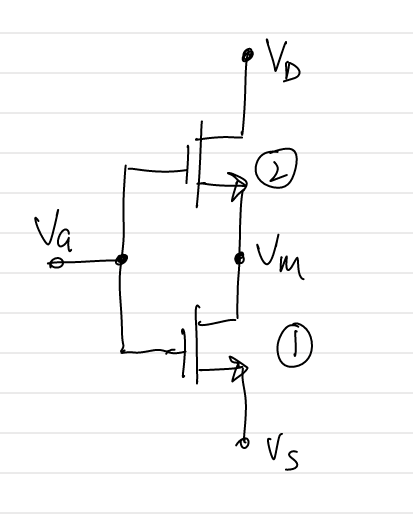# 2 FET in Series# Circuit [top]

The following circuit is analyzed:# Derivation [top]

The above circuit will always have transistor 1 in triode operation because:
`\$\$V_{MS}=V_{M}-V_{S}<V_{GS}-V_{TH}\$\$`

This condition is needed for transistor 2 to be above cut off.
With this condition we have:
`\$\$I_{DS} = I_{DS1} = I_{DS2}........(1)\$\$`

`\$\$I_{DS1}=μ_nC_{OX}W/L[(V_{GS}-V_{TH})V_{MS}-{{V_{MS}}^2}/2].........(2)\$\$`

`\$\$I_{DS2}=1/2μ_nC_{OX}W/L{(V_{GM}-V_{TH})}^2\$\$`

Also:
`\$\$V_{GM}=V_{GS}-V_{MS}\$\$`

So we have:
`\$\$I_{DS2}=1/2μ_nC_{OX}W/L{(V_{GS}-V_{TH}-V_{MS})}^2\$\$`

`\$\$I_{DS2}=1/2μ_nC_{OX}W/L[{(V_{GS}-V_{TH})}^2-V_{MS}^2-2V_{MS}(V_{GS}-V_{TH})]\$\$`

From (1) we have:
`\$\$1/2μ_nC_{OX}W/L[{(V_{GS}-V_{TH})}^2+V_{MS}^2-2V_{MS}(V_{GS}-V_{TH})]=μ_nC_{OX}W/L[(V_{GS}-V_{TH})V_{MS}-{{V_{MS}}^2}/2]\$\$`

`\$\${(V_{GS}-V_{TH})}^2/2+V_{MS}^2/2-V_{MS}(V_{GS}-V_{TH})=(V_{GS}-V_{TH})V_{MS}-{{V_{MS}}^2}/2\$\$`

`\$\$⇒{(V_{GS}-V_{TH})}^2/4=(V_{GS}-V_{TH})V_{MS}-{{V_{MS}}^2}/2\$\$`

Put this in (2) we have
`\$\$I_{DS1}=1/2μ_nC_{OX}W/{2L}{(V_{GS}-V_{TH})}^2\$\$`

Hence having 2 FET in series is equivalent to approximately having a single transistor with twice the length or half the width. Approximate because we did the derivation assuming that transistors comply with the square law equation everywhere and because we neglected any body effect that may happen on transistor 2.

# Usage [top]

So the question is why would you need to use this and why not have a single transistor with 2 times the length or half the width?
There are a couple of reasons where you may want to use such a configuration:
1. If in a current mirroring scheme you want to generate a small current. Suppose you have a mirror that generates 1uA and higher multiples so you designed the cascode transistor or mirror to work with 1uA as 1x size (W and L). Now in your circuit you need 0.5uA. To do that if you use a 0.5x (0.5W and L or W and 2L) this changes the transistors characteristics unpredictably because of different W and L than the main mirroring array. Also this totally different size will be difficulat to incorporate in a neat layout with common centroid and uniform placement. It will be much better to use 2 transistors in series in this case. The other option would have been to make this a 1x size and everything else in the mirroring structure would double in size to make everything integer multiples.
2. The other reason is speed and capacitance. Suppose you have a mirror with cascode designed for a particular current. You can design the cascode with 2 transistors in series so for a double current mirror your cascode would just have 1 transistor and hence low capacitance on the current output node instead of the alternative where you have 1 transistor in the current input and 2 transistors in the current output.